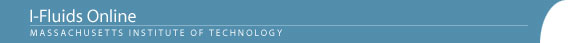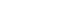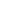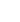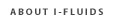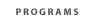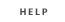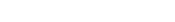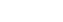2.25: Advanced Fluid Dynamics Section 1: Continuum viewpoint and the equation of motion Section 2: Static Fluids Section 3: Mass Conservation Section 4: Inviscid flow - differential approach Section 5: Control Volume Theorums Section 6: Navier-Stokes equation and viscous flow Section 7: Similarity and dimensional analysis Section 8: Boundary layers, separation and effect on drag/lift Section 9: Vorticity and circulation Section 10: Potential flows; lift, drag and thrust production Section 11: Surface tension and its effect on flows Section 12: Introduction to turbulence Back to 2.25 HomeSection 5: Control Volume Theorums5.1 Physical laws expressed first in terms of a material particle and then in terms of a material volume - a closed system that consists always of the same material particles: law of mass conservation, equation of motion, first law of thermodynamics, second law of thermodynamics.
5.2 The Reynolds transformation, which allows the physical laws that apply to material volumes to be re-expressed so they apply to an arbitrarily specified control volume æan open system with a completely arbitrarily specified boundary.
5.3 The mass conservation law applied to a control volume. Examples involving various control volume choices for a given problem.
5.4 The linear momentum equation (Newton's law of motion in control volume form). Various examples, steady and unsteady, including a fuel-laden space rocket accelerating to speed, transient flows involving phase change (and the reaction stress that causes), examples from hydraulics and surface waves, determining drag from a wake profiles, etc.
5.5 The angular momentum theorem (a moment of Newton's law, expressed in control volume form). Various examples, ranging from lawn sprinklers and centrifugal pumps to the transient spin-up of bathtub vortices and tornadoes.
5.6 The energy equation (first law of thermodynamics) and the second law of thermodynamics in control volume form.